Question

# COI) (15 pts) Two capacitors, C and C2, are connected in series to a 60 V battery as shown in the sketch in part b). The capacitance of C, is 1.0 x10 F, and it carries a charge of2.0 10 C. R-100 ohms...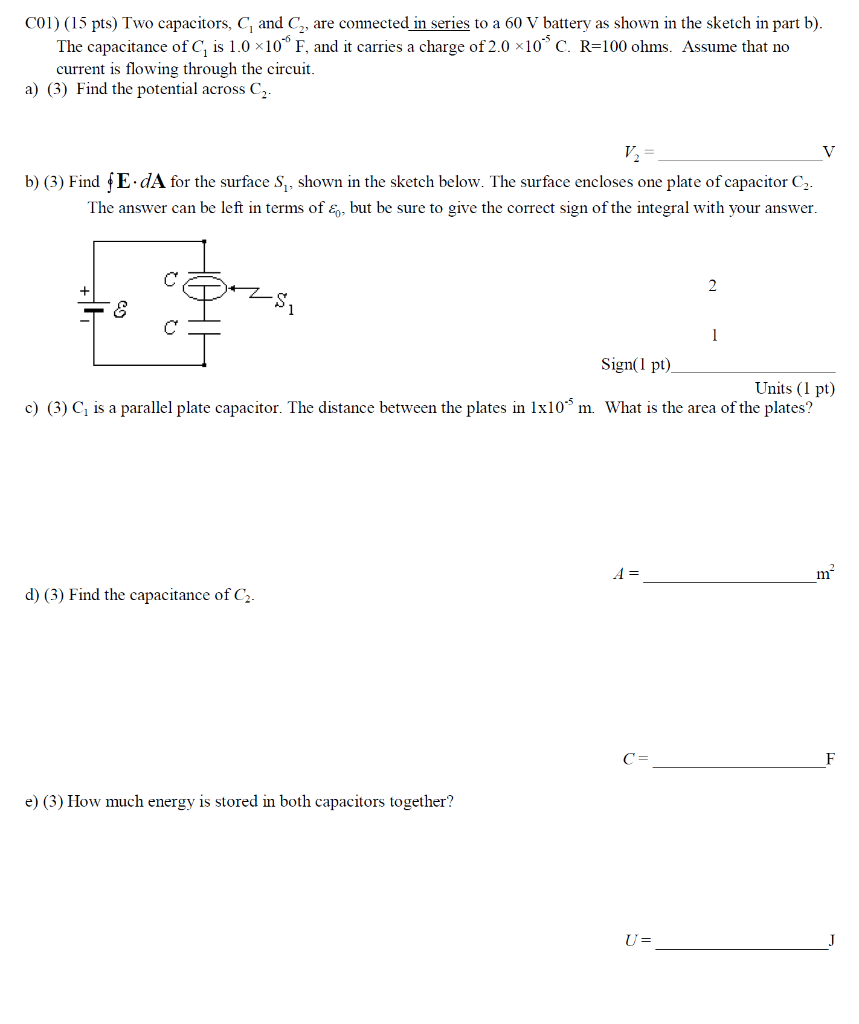COI) (15 pts) Two capacitors, C and C2, are connected in series to a 60 V battery as shown in the sketch in part b). The capacitance of C, is 1.0 x10 F, and it carries a charge of2.0 10 C. R-100 ohms. Assume that no current is flowing through the circuit. a) () Find the potential across C b) (3) Find fE dA for the surface S, shown in the sketch below. The surface encloses one plate of capacitor C2 The answer can be left in terms of &, but be sure to give the correct sign of the integral with your answer. Sign(l pt) Units (1 pt) c) (8) C, is a parallel plate capacitor. The distance between the plates in 1x10* m What is the area of the plates? d) (3) Find the capacitance of C2 e) (3) How much energy is stored in both capacitors together?

Summary - it is basic problem so I have shown step by step solution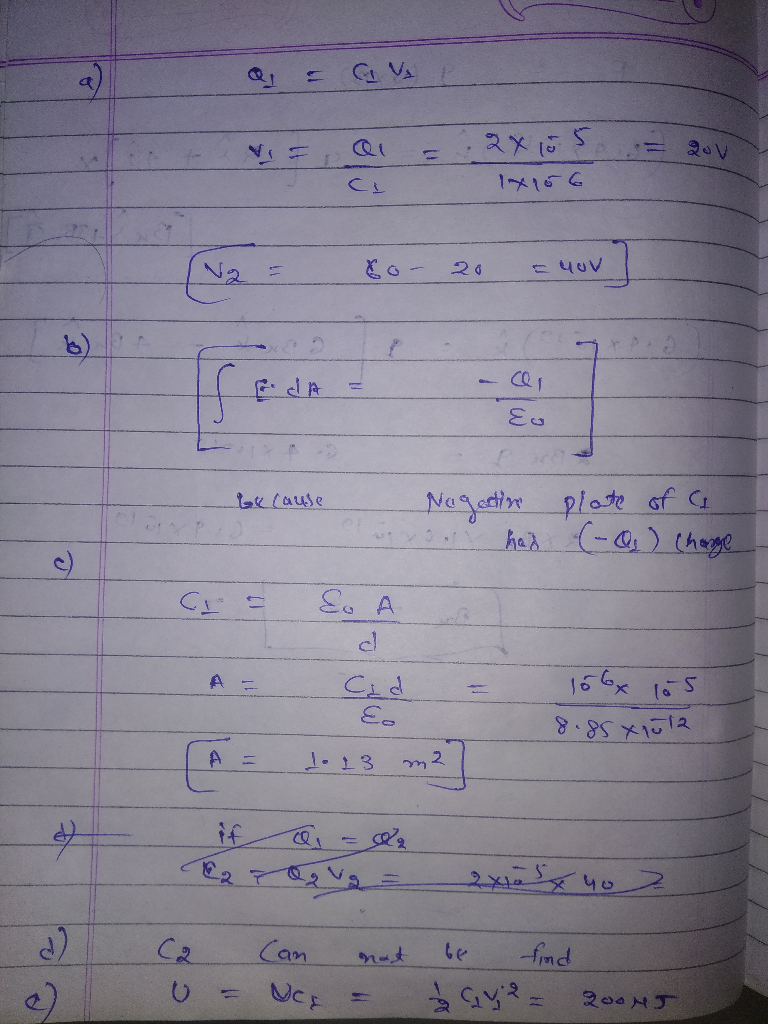#### Earn Coins

Coins can be redeemed for fabulous gifts.

Similar Homework Help Questions
• ### Two capacitors, C119.0 F and C2 32.0 uf are connected in series, and a 9.0-V battery...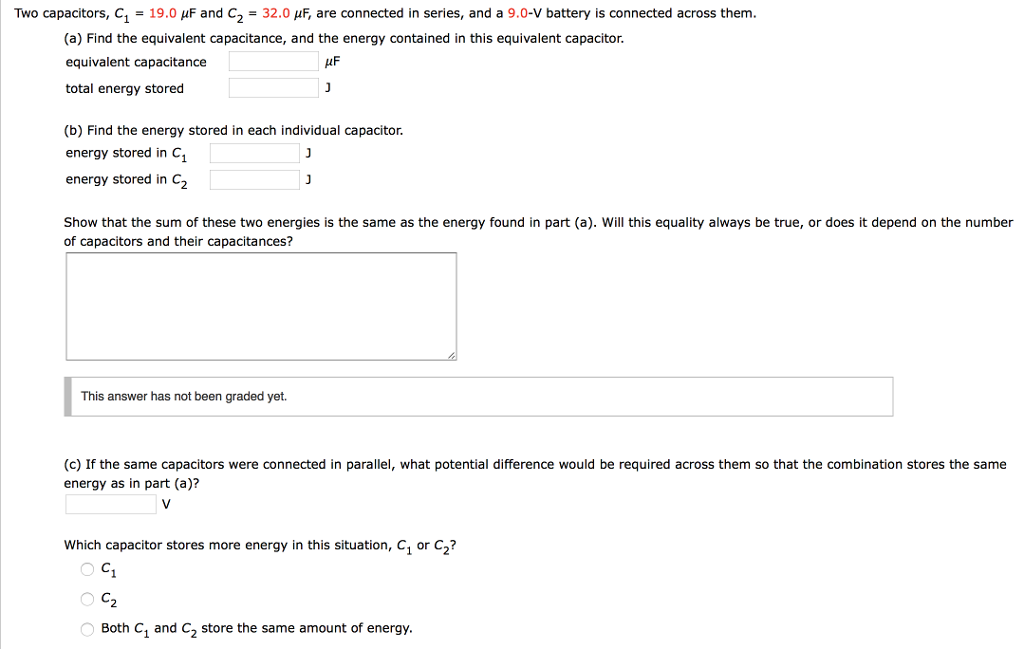Two capacitors, C119.0 F and C2 32.0 uf are connected in series, and a 9.0-V battery is connected across them (a) Find the equivalent capacitance, and the energy contained in this equivalent capacitor. equivalent capacitance total energy stored (b) Find the energy stored in each individual capacitor. energy stored in C1 energy stored in C2 Show that the sum of these two energies is the same as the energy found in part (a). Will this equality always be true, or...

• ### Two capacitors, C1 = 26.0 μF and C2=37.0 μF, are connected in series, and a 9.0-v battery is connected across them.

Two capacitors, C1 = 26.0 μF and C2=37.0 μF, are connected in series, and a 9.0-v battery is connected across them. (a) Find the equivalent capacitance, and the energy contained in this equivalent capacitor(b) Find the energy stored in each individual capacitor(c) If the same capacitors were connected in parallel, what potential difference would be required across them so that the combination stores the same energy as in part (a)? Which capacitor stores more energy in this situation, C1 or C2?

• ### Two capacitors, C 19.0 HF and C2 40.0 HF, are connected in series, and a 21.0-V...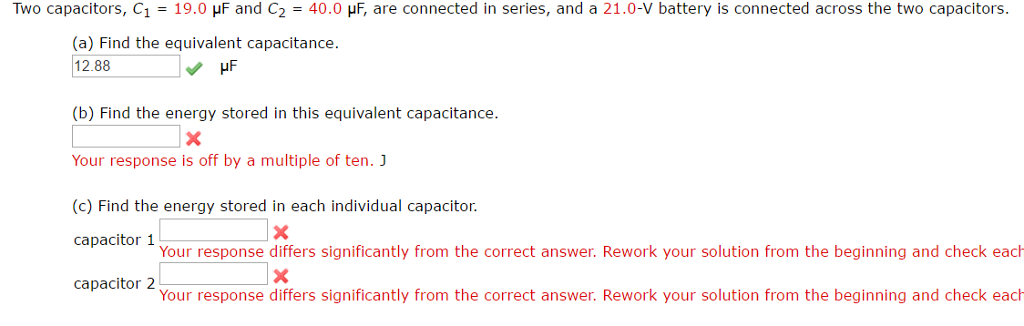Two capacitors, C 19.0 HF and C2 40.0 HF, are connected in series, and a 21.0-V battery is connected across the two capacitors (a) Find the equivalent capacitance. 12.88 v HF (b) Find the energy stored in this equivalent capacitance. Your response is off by a multiple of ten. J (c) Find the energy stored in each individual capacitor. capacitor 1 Your response differs significantly from the correct answer. Rework your solution from the beginning and check each capacitor 2...

• ### Two capacitors, C1-24.0 μF and C2-41.0 μF, are connected in series, and a 21.0-V battery is...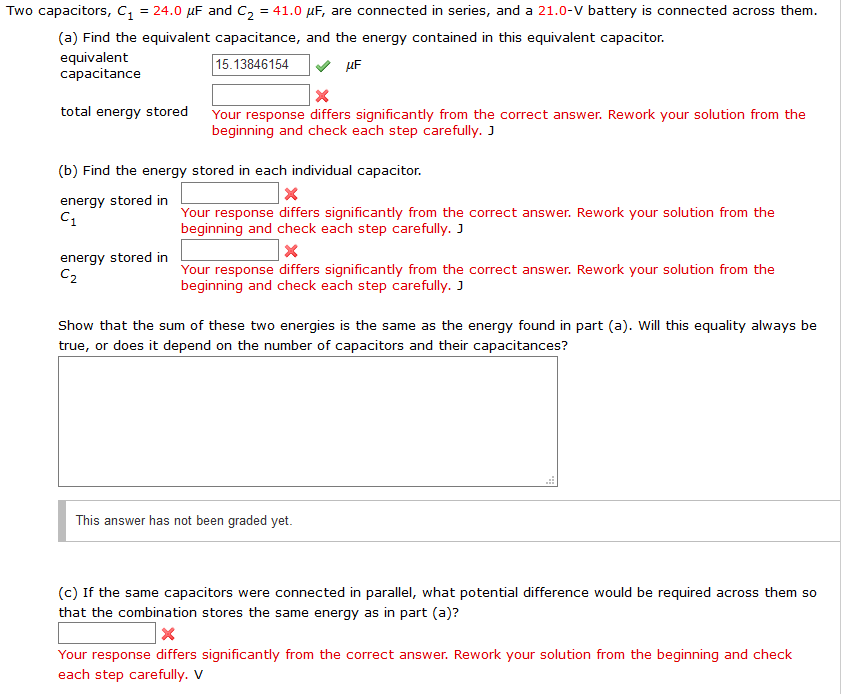Two capacitors, C1-24.0 μF and C2-41.0 μF, are connected in series, and a 21.0-V battery is connected across them (a) Find the equivalent capacitance, and the energy contained in this equivalent capacitor. equivalent capacitance 15.13846154F total energy storedYour response differs significantly from the correct answer. Rework your solution from the beginning and check each step carefully. J (b) Find the energy stored in each individual capacitor energy stored in Your response differs significantly from the correct answer. Rework your solution...